Homework Help Question & Answers

# A river shown in (Figure 1) has an average width of 5 m. Just after its...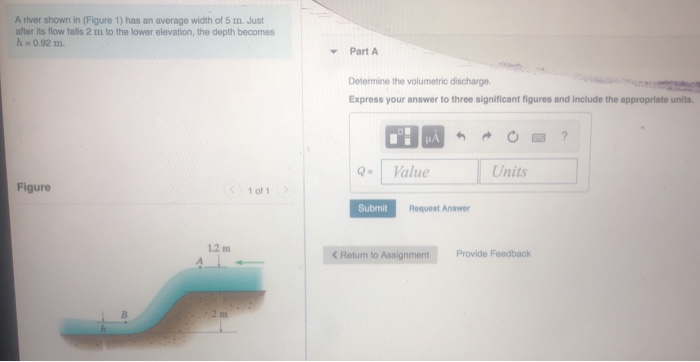A river shown in (Figure 1) has an average width of 5 m. Just after its flow falls 2 m to the lower elevation, the depth becomes h = 0.92 m Part A Determine the volumetric discharge. Express your answer to three significant figures and include the appropriate units. Value Units Figure 101 Submit Request Answer 1.2 m < Return to Assignment Provide Feedback

#### Homework Answers

Answer #1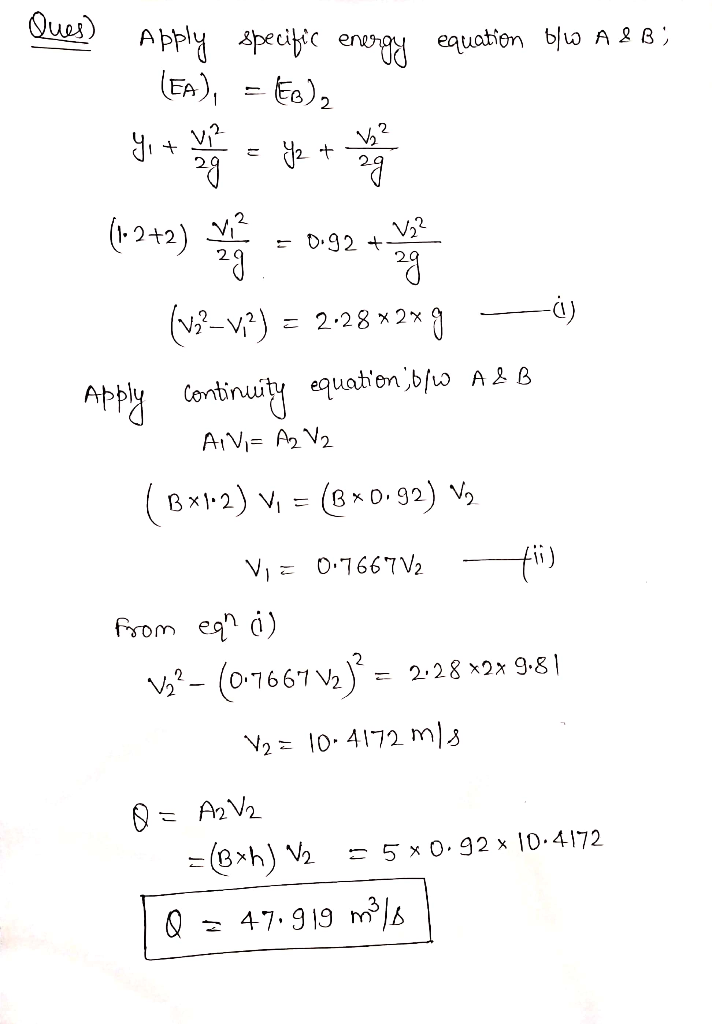please rate

Know the answer?
Your Answer:

#### Post as a guest

Your Name:

What's your source?

#### Earn Coin

Coins can be redeemed for fabulous gifts.

Not the answer you're looking for? Ask your own homework help question. Our experts will answer your question WITHIN MINUTES for Free.
Similar Homework Help Questions
• ### Consider the gate shown in (Figure 1). The gate has a width of 1 m. pw...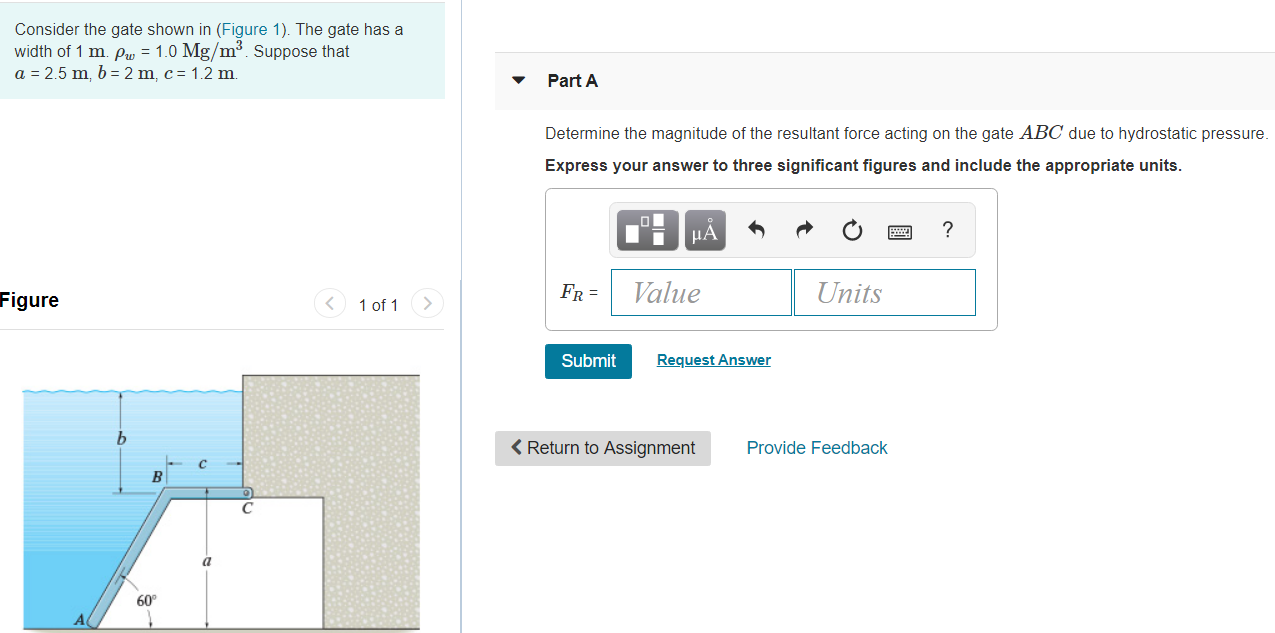Consider the gate shown in (Figure 1). The gate has a width of 1 m. pw = 1.0 Mg/m Suppose that a = 2.5 m, b= 2 m. c= 1.2 m Part A Determine the magnitude of the resultant force acting on the gate ABC due to hydrostatic pressure. Express your answer to three significant figures and include the appropriate units. MÅN O ? Figure FR = Value Units < 1 of 1 > Submit Request Answer < Return to...

• ### Problem 12.83 The rectangular channel has a width of b = 2.5 m and the depth...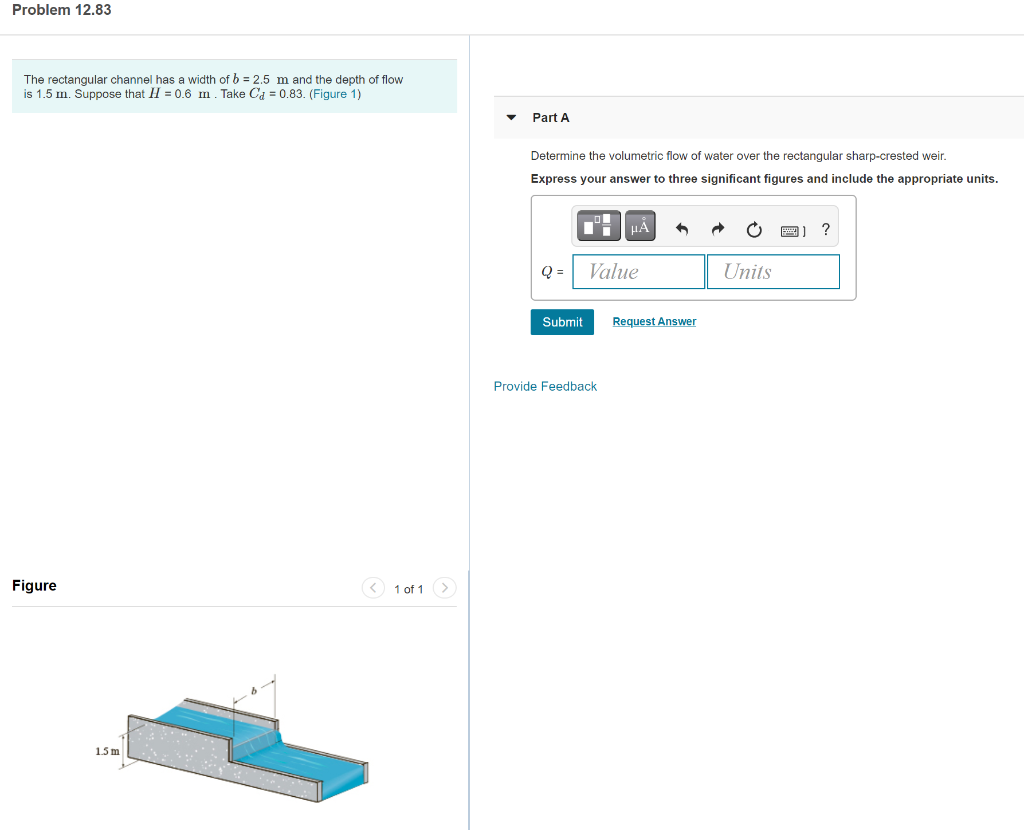Problem 12.83 The rectangular channel has a width of b = 2.5 m and the depth of flow is 1.5 m. Suppose that H = 0.6 m . Take Cd = 0.83. (Figure 1) Part A Determine the volumetric flow of water over the rectangular sharp-crested weir. Express your answer to three significant figures and include the appropriate units. HA ? Value Units Submit Request Answer Provide Feedback Figure < 1 of 1 1.5 m

• ### Item 8 The rectangular channel has a width of 8 ft and transports water at 18(103)...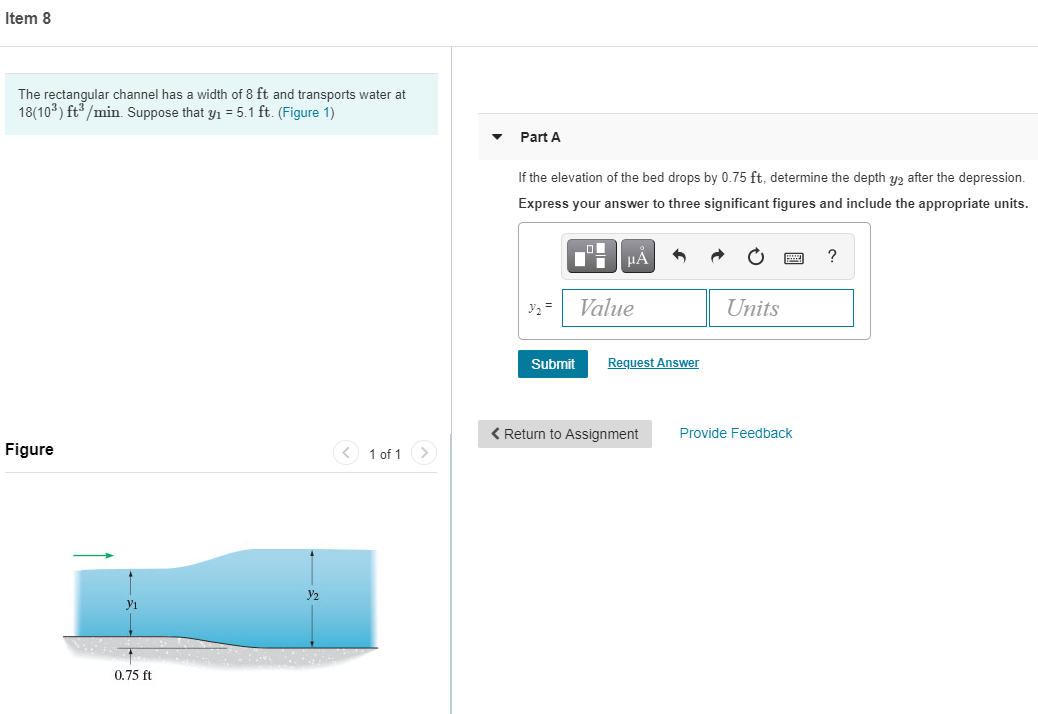Item 8 The rectangular channel has a width of 8 ft and transports water at 18(103) ft3/min. Suppose that yı = 5.1 ft. (Figure 1) Part A If the elevation of the bed drops by 0.75 ft, determine the depth y2 after the depression. Express your answer to three significant figures and include the appropriate units. НА ? Value Units Submit Request Answer < Return to Assignment Provide Feedback Figure < 1 of 1 > Y2 0.75 ft

• ### Part A Water runs from a sloping channel with a flow of 21 m/s onto a...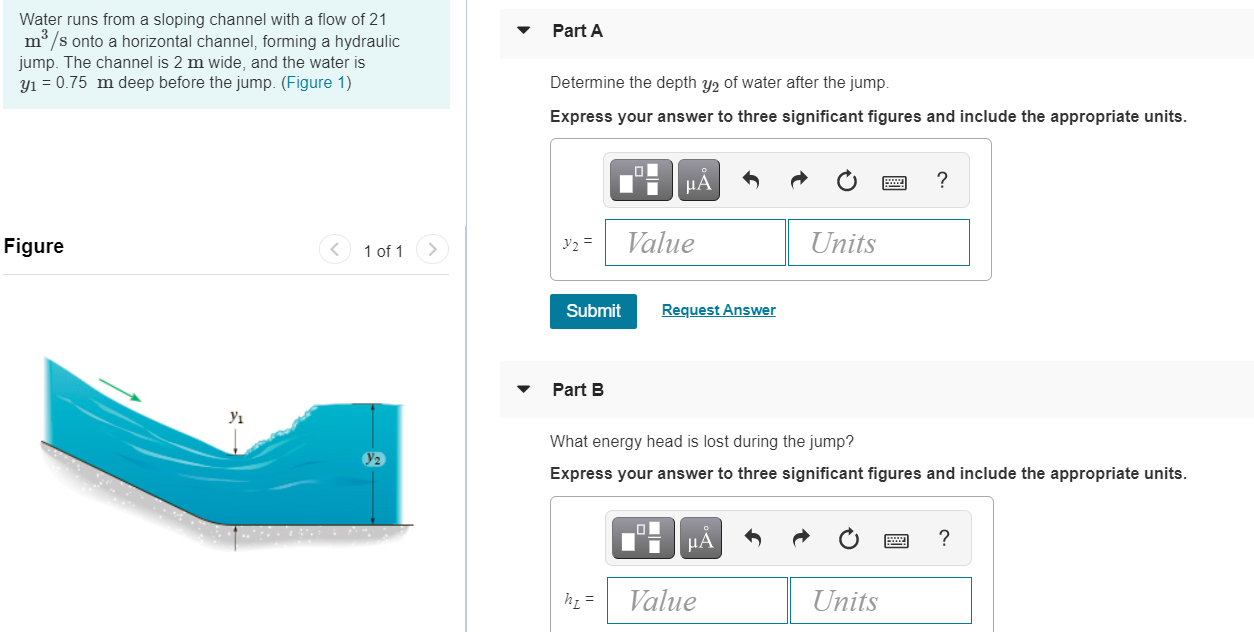Part A Water runs from a sloping channel with a flow of 21 m/s onto a horizontal channel, forming a hydraulic jump. The channel is 2 m wide, and the water is y1 = 0.75 m deep before the jump. (Figure 1) Determine the depth Y2 of water after the jump. Express your answer to three significant figures and include the appropriate units. μΑ ? Figure y2 = Value 1 of 1 Units Submit Request Answer Part B Y2 What...

• ### The drainage pipe is made of finished concrete and is sloped downward at 0.002. (Figure 1)...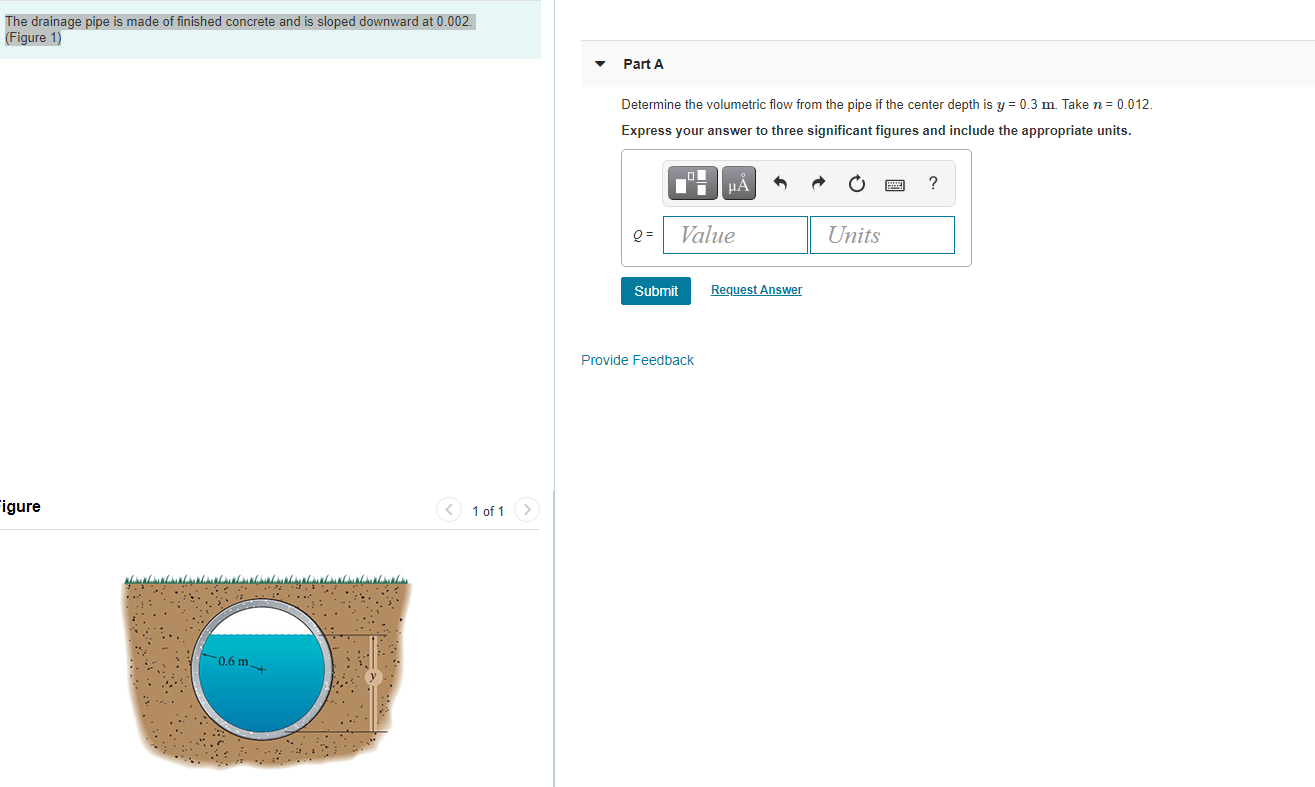The drainage pipe is made of finished concrete and is sloped downward at 0.002. (Figure 1) Part A Determine the volumetric flow from the pipe if the center depth is y = 0.3 m. Take n = 0.012 Express your answer to three significant figures and include the appropriate units. uÅ ? Value Units Submit Request Answer Provide Feedback Figure < 1 of 1 -0.6 m

• ### Problem 12.48 Review Part A The drainage pipe sloped downward at 0.002. (Figure 1) finished concrete...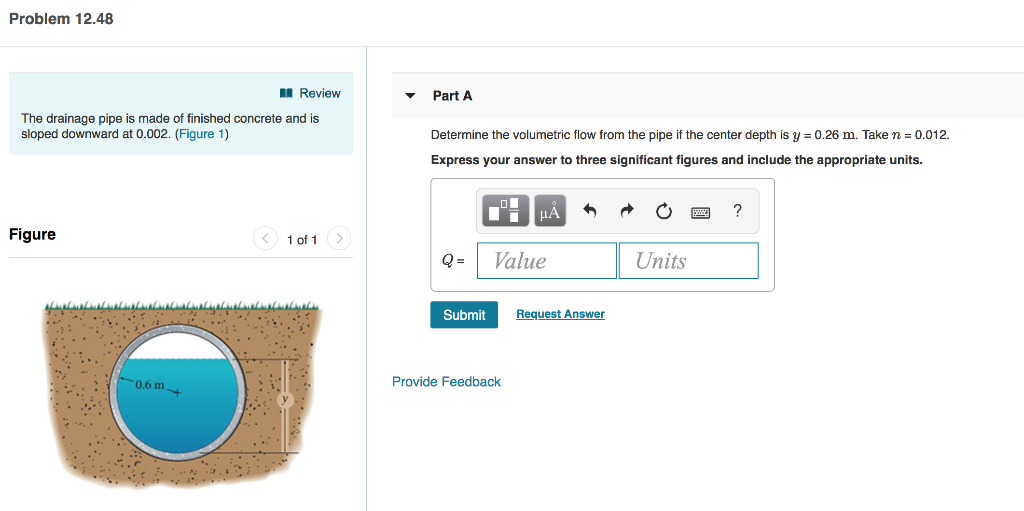Problem 12.48 Review Part A The drainage pipe sloped downward at 0.002. (Figure 1) finished concrete and is made Determine the volumetric flow from the pipe if the center depth is y 0.26 m. Take n 0.012. Express your answer to three significant figures and include the appropriate units. Figure 1 of 1 Value Units Q = Request Answer Submit Provide Feedback 0.6 m

• ### Item 3 3 of 8 The rectangular channel has a width of 8 ft and transports...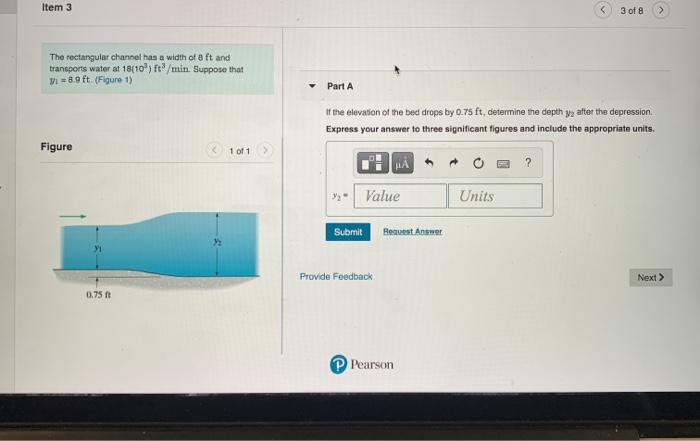Item 3 3 of 8 The rectangular channel has a width of 8 ft and transports water at 18(10) ft/min. Suppose that Vi = 8.9 ft. (Figure 1) Part A If the elevation of the bed drops by 0.75 ft, determine the depth ya after the depression Express your answer to three significant figures and include the appropriate units. Figure < 1 of 1 H ? Value Units Submit Request Answer Provide Feedback Next > 0.75 P Pearson

• ### The channel transports water at 6 m/s. The depth of flow is y = 1.5 m...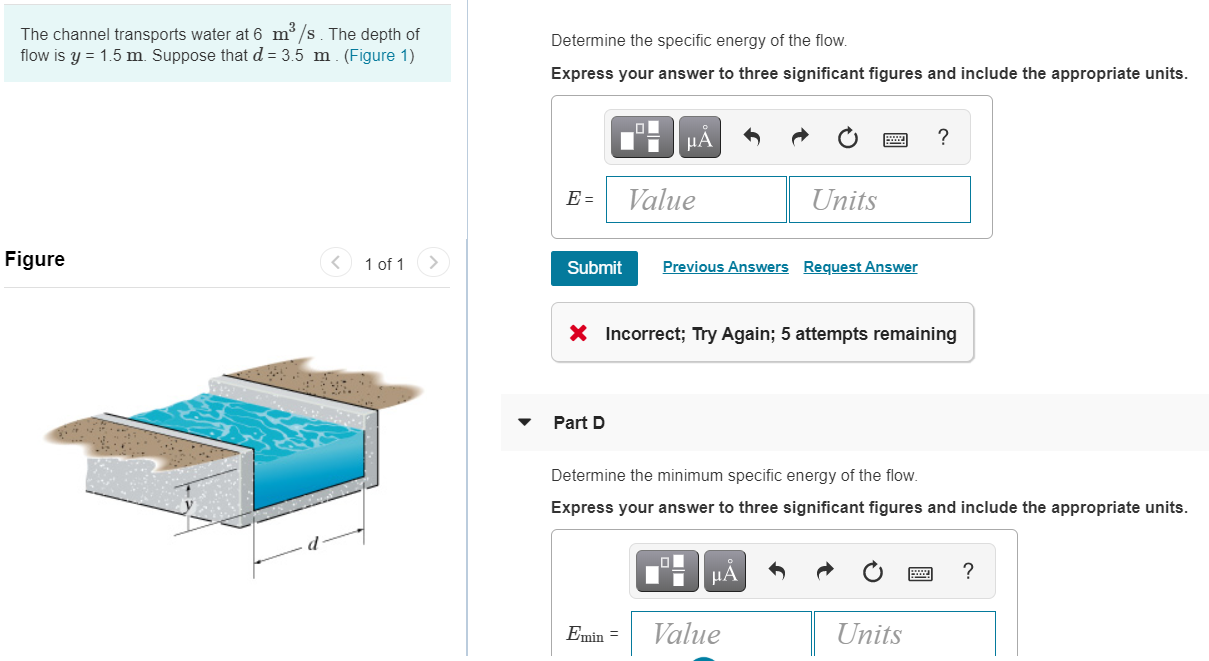The channel transports water at 6 m/s. The depth of flow is y = 1.5 m Suppose that d = 3.5 m (Figure 1) Determine the specific energy of the flow. Express your answer to three significant figures and include the appropriate units. μΑ ? E- Value Units Figure < 1 of 1 Submit Previous Answers Request Answer X Incorrect; Try Again; 5 attempts remaining Part D Determine the minimum specific energy of the flow. Express your answer to three...

• ### The flow of water over the broad-crested weir is 15 m3/s. The weir and the channel...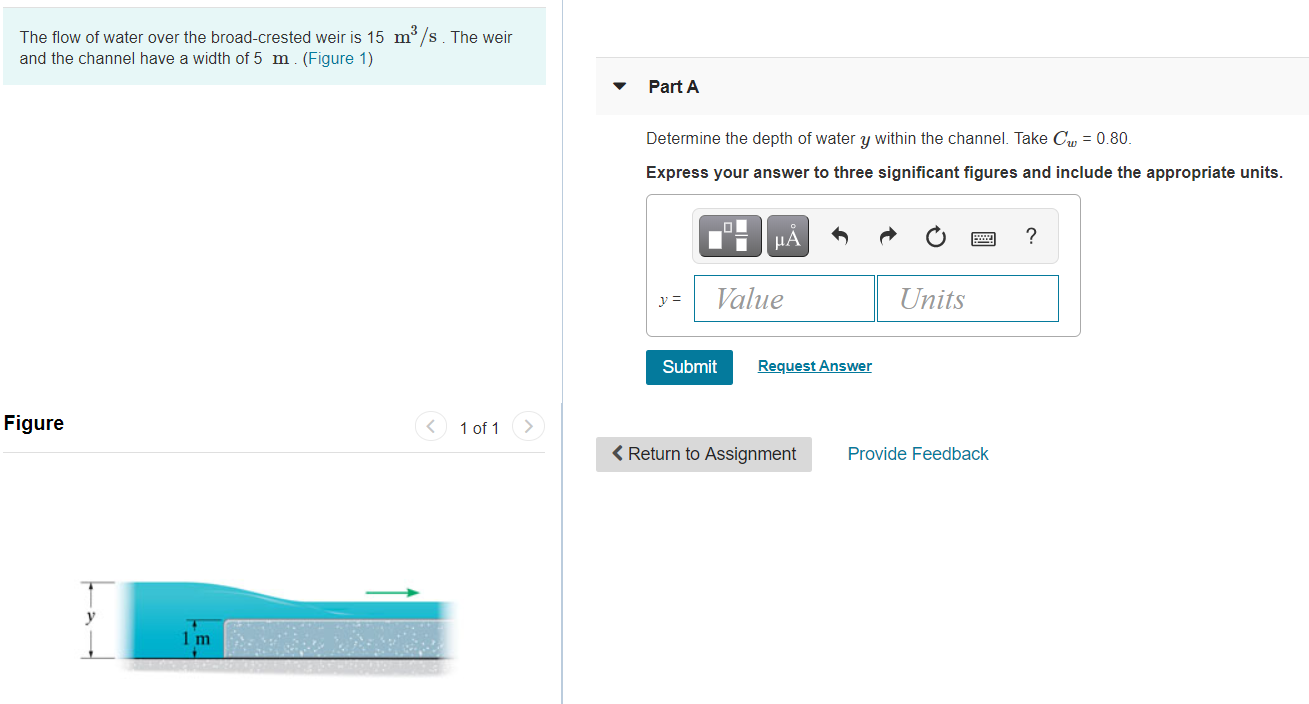The flow of water over the broad-crested weir is 15 m3/s. The weir and the channel have a width of 5 m. (Figure 1) Part A Determine the depth of water y within the channel. Take Cw = 0.80. Express your answer to three significant figures and include the appropriate units. μΑ ? y = Value Units Submit Request Answer Figure < 1 of 1 < Return to Assignment Provide Feedback 1 m Water flows at 450 ft3/s over the...

• ### Item 2 2 The rectangular channel has a width of 8 ft and transports water at...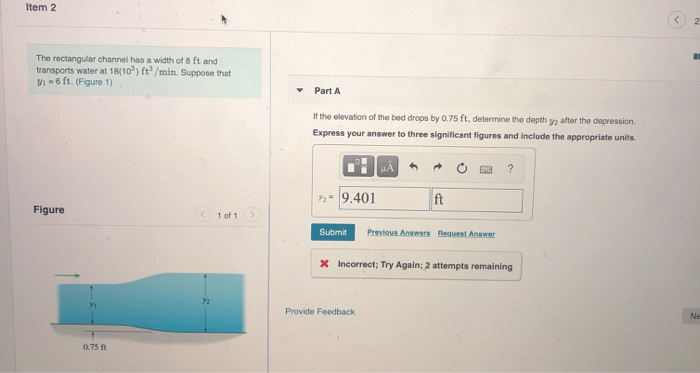Item 2 2 The rectangular channel has a width of 8 ft and transports water at 18(10) ft/min. Suppose that Vi = 6 ft. (Figure 1) Part A I the elevation of the bed drops by 0.75 ft, determine the depth y after the depression Express your answer to three significant figures and include the appropriate units. MA ? Y. - 9.401 ft Figure (1 of 1 Submit Previous Answers Request Answer X Incorrect; Try Again: 2 attempts remaining Provide...